# Cam forces acting on a linear motion bearing with nonlinear forces

• Jeremy Sawatzky
In summary, the press force comes from a roller bearing mounted to a piston shaft, the rod sliding through a rigid linear bearing and the piston on the end of the shaft acting on the product. The roller bearing is rolling on a surface that gradually slopes downward imparting a downward force but also a sideways force on the piston rod and as such the linear bushing. The forces all originate from the bushing. The bushing imparts the side force onto the piston rod. The sloped surface that the roller rides on is mounted rigidly. There are 120 pistons on top and another 120 on the bottom. 1 cam follower acts upon 3 pistonsf

#### Jeremy Sawatzky

TL;DR Summary
I have a cam roller on a sloped surface providing a pressing force to a product. How aggressive can my cam surface be without destroying the linear bearing prematurely?
I have a machine I am designing that for all intensive descriptions, is a simple press designed to compress loose product into a puck like shape.

The press force comes from a roller bearing mounted to a piston shaft, the rod sliding through a rigid linear bearing and the piston on the end of the shaft acting on the product. The roller bearing is rolling on a surface that gradually slopes downward imparting a downward force but also a sideways force on the piston rod and as such the linear bushing.

My goal is to determine how aggressive of a slope I can have the roller bearing interacting with. as the slope angle increases, so do the side forces on the piston shaft, so do the forces on the bushing. I recognize that I am dealing with point pressures on the top and bottom edges of the bushing.

Lastly, the forces all originate from the bushing. The bushing imparts the side force onto the piston rod. The sloped surface that the roller rides on is mounted rigidly.

Thank you!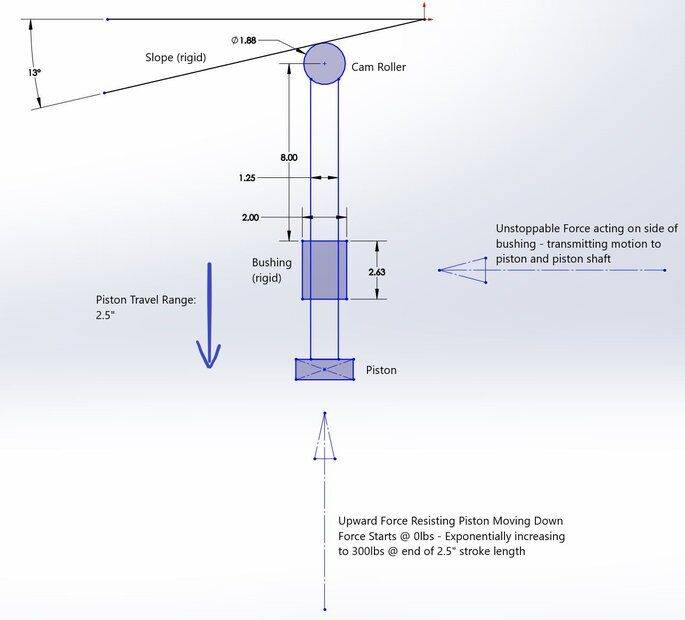Could you use two linear bearings rather that one?
If so, the increased distance between then would help decrease the top/bottom point pressures.

Actually yes I can and I have been looking for solutions. I found these https://www.igus.ca/product/391?artNr=EGLM-30

Having 2 self aligning bearings eliminates the edge loading. But regardless I want to know the math behind the setup.

I do not like the short bushing, 2.63” with the long 8” shaft to the roller. That places significant forces on the edges of the bushing. I would look for a way of rotating that shaft and bush by 13° which would make it perpendicular to the cam wedge surface. The implications for the piston below are not shown in your diagram.

Alternatively, a rocker would make a more reliable mechanism to couple the roller to the piston. Indeed, a linkage might eliminate the roller and the 13° taper. At the same time a two links forming a 'knee' could straighten, largely compensating for the exponentially increasing force required as the piston is pressed.

There is no room for that unfortunately even though its huge, probably about 6000 lbs.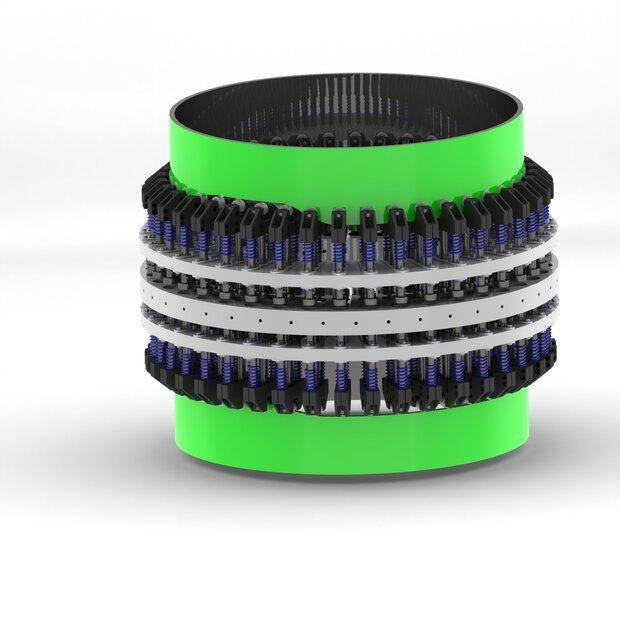Last edited:
I have a machine I am designing that for all intensive descriptions, is a simple press designed to compress loose product into a puck like shape.
This sentence makes more sense when I replace "intensive descriptions" with "intents and purposes".

The word "intensive" is becoming a very common malapropism for "intents" in the phrase "for all intents and purposes". Once it has been pointed out, one who cannot change their use become a "mumpsimus". Words can be so much fun.

There are many examples; "Being specific" means something completely different to "being pacific". It is February, not Febuary.

This is much like the machines used to manufacture flash light batteries. They too use a number of cams in a circular array to pack the material.

If you ramp is truly a straight line, as you have indicated, this is an extremely simple problem to analyze because the pressure angle is constant. If there is actually a curved ramp involved (as would be much more common), then it is necessary to determine the pressure angle at each position. With the pressure angle and load known, the forces can all be calculated. Then you simply need to find a bearing geometry that can withstand those loads.

Yes I just need to know how to calculate for simple angles. Then I can play with different paths and angles to get the job done. I have the constraints, just need the math portion.

If I understand the image in post #5 correctly, there is another (larger) problem.

It looks like the cam followers are at a different radial distance than the return spring and the working plunger. With the downward force from the cam not axial to the 300lb plunger reaction force, there is a rather large force trying to tip the plungers toward the cam (radially inward).

Cheers,
Tom

•Lnewqban
There are 120 pistons on top and another 120 on the bottom. 1 cam follower acts upon 3 pistons at once.

The math is really all I need. How much side loading is occurring at the bushing location relative to the 300# resistance to the piston moving down.

The math is really all I need. How much side loading is occurring at the bushing location relative to the 300# resistance to the piston moving down.
I would expect the side-force Fs, to be the down-force Fd, multiplied by the tangent of the wedge-angle, θ = 13° in your case; Tan( 13° ) = 0.231
Fs = Fd * tan( θ )

Alright so that would be about 70ish lbs side force. - So how does that relate to my distance from the bushing? I need to multiple over the distance or something? I think what I actually need to do is take the distance from the center of the track roller to the top of the bushing divided by the length of the bushing and I multiply by 70#.

- I have since changed the bushing design. now I am using 2 self aligning bearings on top of each other. - the self aligning bearing (2 of them) removes the edge loading and applies this load to the whole bearing.

So my math equates to 477 lbs side load on each bearing. - each bearing is rated for 900 so that's good.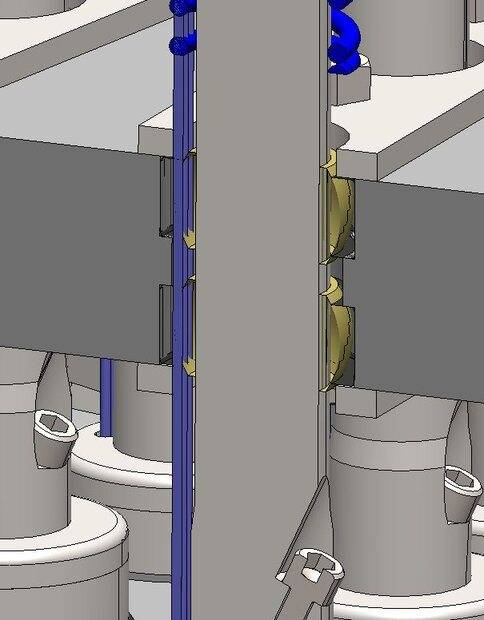Please anyone let me know if this is way off. Cheers!

Last edited:
- I have since changed the bushing design. now I am using 2 self aligning bearings on top of each other. - the self aligning bearing (2 of them) removes the edge loading and applies this load to the whole bearing.
In effect it applies the average force at the mid point of each of the two spherical bearings, so the distance between the bearings is now about half the sleeve bearing length. The ability for the bearing to align with the shaft is a big advantage.

If you could bore the holes in the rotor at the same angle as the cam slope, there would be no side force during compression. An alternative would be to push in a sleeve bearing that had been bored diagonally, at the cam angle.

Cant do that, has to be perpendicular. The cammed portion of the machine only lasts for about 20 degrees of the rotation. The rest is just holding the product in the pressed state.

Yes the mid point between the 2 bearings is only about 1.1" I may increase the thickness of the plate a further 1/2" to increase the distance.

I have also begun detailing a cam follower that acts in reverse of the compression. Lifting instead of lowering. This removes the needs for springs which allows me to shorten the piston shafts - further aiding the geometry of the bushing loading

As a follow up to this post, I have done some real world testing and found the actual force to be about 3000lbs to compress this product. The self aligning bushings are rated for 2700lbs of side force each.

I have attached a new diagram showing the latest data.

The force on the piston assembly is shown. As the Cam Roller moves through its positions, it begins to encounter resistive force starting at 0lbs moving up to 3000lb though the last 0.48" downward travel of the cam profile.

The information I am looking for is as the cam roller is being pushed into an 18.75° sloped surface, what is the force (lbs) that is being imparted onto the cam roller itself and secondly, given that the bushings are 1.64" apart, what is the side load on the bushings?

Thanks for any and all help.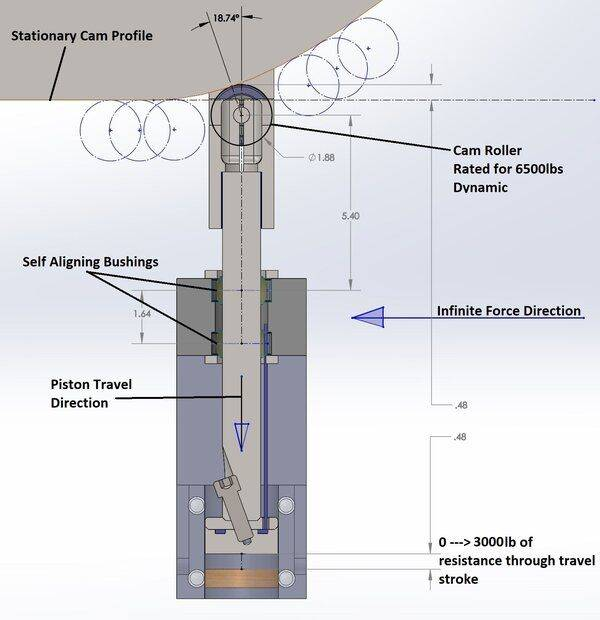To calculate bearing forces, we start with a free body diagram (FBD). A simple FBD to get started is shown below: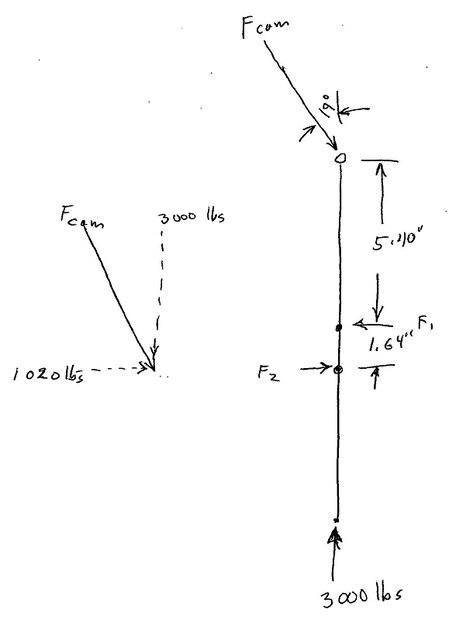In an FBD, all forces are shown on a stick diagram. Unknown forces are solved for using the principle that all forces and all moments sum to zero.

We know the force at the end, 3000 lbs. We start with the simplifying assumption that the linear bearings have zero friction. The cam force is at an 18.7 degree angle, and the vertical component of the cam force is 3000 lbs. The vertical force components now sum to zero. We calculate the cam force, ##F_{cam}##, using trigonometry: ##F_{cam} = 3000 /\cos(18.74) = 3170 lbs##. That is the total force on the cam.

The horizontal component of the cam force is ##F_{cam horz} = 3170 * \sin(18.74) = 1020 lbs##. The linear bearing forces are calculated from the horizontal component of the cam force. One way to start (not the only way) is to calculate the sum of moments about the lower linear bearing. The vertical force components act through the center of that bearing, so their moments are zero. The horizontal cam force component has a moment of ##M = 1020 lbs * 7.04 inches = 7160 in-lbs##. Force ##F_1## has an equal and opposite moment, so is ##F_1 = 7160 / 1.64 inches = 4370 lbs##. Force ##F_2## is calculated by summing horizontal forces to zero: ##F_2 = 4370 - 1020 = 3050 lbs##.

There is one more step. We need to include the effects of friction in the linear bearings. Friction in the linear bearings adds to the 3000 lb load force, and makes the cam force even larger than calculated above. Physicists and engineers calculate the added friction force by creating a system of equations, and solving. In your case, we can use an easier to understand, but tedious, method that gets the job done.

Given the first estimate of linear bearing forces from above, and a coefficient of friction, we estimate the friction force from each bearing. Choosing the friction coefficient to be 0.1, the friction force from the top linear bearing is ##F_{1fric} = 0.1 * 4370 = 437 lbs##. Similarly,##F_{2fric} = 0.1 * 3050 = 305 lbs##. Then the vertical component of the cam force is ##3000 + 437 + 305 = 3740 lbs##. Now repeat the calculations for total cam force, horizontal component of the cam force, and linear bearing force. Go through this cycle until the cam force stops changing.

These calculations can be tedious, but they are necessary. If the increase in cam force is smaller at each step, the calculations are converging on the true cam force. If the increase in cam force is larger at each step, then the combination of cam angle, distance to the linear bearings, linear bearing spacing, and coefficient of friction is self locking. In that case, the machine will destroy itself. The calculations also show that the cam force will always be larger than the load force, and that the linear bearing forces can easily be very large. Note also that the horizontal component of the cam force is the force to drive this mechanism.

This is outstanding, thanks very much for the detailed response. So if I understand correctly, given that even before including friction losses in the calculations, F1 is 4370lbs and F2 is 3050 lbs, my bearings (rated at 2700lbs) each will not withstand the forces.

With that information my first objective should be to reduce the distance from the cam roller to the linear bearings.

Adding to the complexity of the math here, I may have assumed my 3000# force as being too high. In this machine, this "press" is actually compressing 3 products at once, each one a small hockey puck shape, each one taking 1000lbs to compress. Am I correct that it is simply a matter of adding up the compressive forces of the 3 products? or (as I have heard) it is not that simple, to compress 1 of something takes x amount of force but to compress 2 of something takes x*1.3 amount of force? (1.3 being a guess) and so on and so on?

My way of thinking makes me think it is infact that simple, 1 = 1000, 2=2000, 3=3000... etc

Am I correct that it is simply a matter of adding up the compressive forces of the 3 products?
No.
How does the puck in the middle know what is above or below?
If the three items are stacked, then the same 1000 lbs can compress all three in the stack.

I should have been more clear, the 3 items are side by side, not stacked on top of one another.

the 3 items are side by side, not stacked on top of one another.
If the three pucks are next to each other, then the area is three times greater.
To achieve the same pressure = force / area; the force needed will be three times greater.